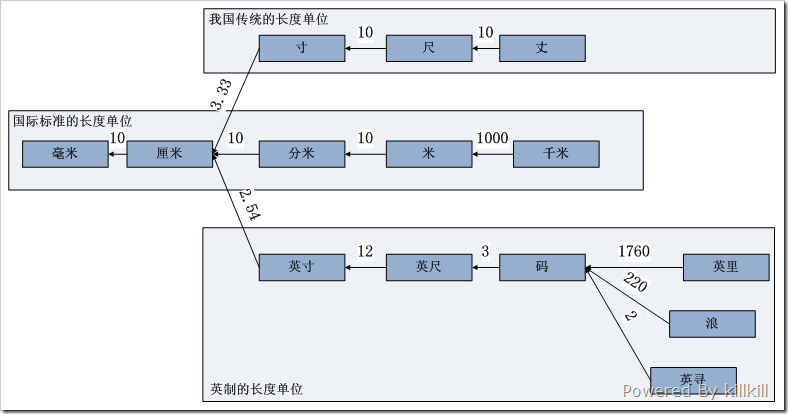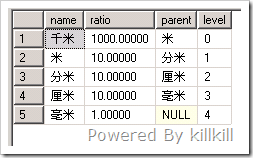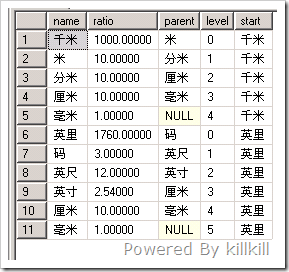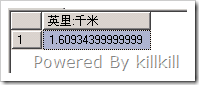# [转载]用SQL做单位换算pumeifen朋友在首页提出了一个问题“SQL 问题 求解”，我对这个问题延伸一下描述为“用数据库来做单位换算”，以长度单位为例，常用的长度单位 有：毫米、厘米、分米等等，而英制的长度单位有英寸、英尺、码等，而我国传统的长度单位也有寸、尺、丈等等。

 `1` `create` `table` `Length`
 `2` `(`
 `3` ` ``name` `varchar2(50),`
 `4` ` ``ratio number(10,5) ``not` `null``,`
 `5` ` ``parent varchar2(50),`
 `6` ` ``constraints pk_length ``primary` `key` `(``name``)`
 `7` `)`

 `01` `insert` `into` `Length `
 `02` `---- 国际标准的长度单位`
 `03` `select` `'毫米'` `,1.0 ,``null` `from` `dual ``union` `all`
 `04` `select` `'厘米'` `,10.0 ,``'毫米'` `from` `dual ``union` `all`
 `05` `select` `'分米'` `,10.0 ,``'厘米'` `from` `dual ``union` `all`
 `06` `select` `'米'` `,10.0 ,``'分米'` `from` `dual ``union` `all`
 `07` `select` `'千米'` `,1000.0 ,``'米'` `from` `dual ``union` `all`
 `08` `---- 我国传统的长度单位`
 `09` `select` `'寸'` `,3.33,``'厘米'` `from` `dual ``union` `all`
 `10` `select` `'尺'` `,10.0 ,``'寸'` `from` `dual ``union` `all`
 `11` `select` `'丈'` `,10.0 ,``'尺'` `from` `dual ``union` `all`
 `12` `---- 少数欧美国家使用的英制长度单位`
 `13` `select` `'英寸'` `, 2.54 , ``'厘米'` `from` `dual ``union` `all`
 `14` `select` `'英尺'` `, 12.0  , ``'英寸'` `from` `dual ``union` `all`
 `15` `select` `'码'` `, 3.0  , ``'英尺'` `from` `dual ``union` `all`
 `16` `select` `'浪'` `, 220.0  , ``'码'` `from` `dual ``union` `all`
 `17` `select` `'英寻'` `, 2.0  , ``'码'` `from` `dual ``union` `all`
 `18` `select` `'英里'` `, 1760.0  , ``'码'` `from` `dual``01` `SELECT` `name``,ratio,parent`
 `02` `FROM` `length`
 `03` `CONNECT` `BY` `nocycle ``PRIOR` `parent = ``name`
 `04` `START ``WITH` `name` `= ``'千米'`
 `05` `union` `all`
 `06` `select` `null``,``null``,``null` `from` `dual`
 `07` `union` `all`
 `08` `SELECT` `name``,ratio,parent`
 `09` `FROM` `length`
 `10` `CONNECT` `BY` `nocycle ``PRIOR` `parent = ``name`
 `11` `START ``WITH` `name` `= ``'英里'`
 `01` `NAME            RATIO PARENT`
 `02` `---------- ---------- ----------`
 `03` `千米             1000 米`
 `04` `米                 10 分米`
 `05` `分米               10 厘米`
 `06` `厘米               10 毫米`
 `07` `毫米                1`
 `08`
 `09` `英里             1760 码`
 `10` `码                  3 英尺`
 `11` `英尺               12 英寸`
 `12` `英寸             2.54 厘米`
 `13` `厘米               10 毫米`
 `14` `毫米                1`

 `01` `select`
 `02` `( ``--英里转化成毫米`
 `03` ` ``select` `exp(``sum``(ln(ratio)))`
 `04` ` ``FROM` `length`
 `05` ` ``CONNECT` `BY` `nocycle ``PRIOR` `parent = ``name`
 `06` ` ``START ``WITH` `name` `= ``'英里'`
 `07` `)/( ``--千米转化成毫米`
 `08` ` ``select` `exp(``sum``(ln(ratio)))`
 `09` ` ``FROM` `length`
 `10` ` ``CONNECT` `BY` `nocycle ``PRIOR` `parent = ``name`
 `11` ` ``START ``WITH` `name` `= ``'千米'`
 `12` `) ``"英里:千米"`
 `13` `from` `dual;`
 `1` `英里:千米`
 `2` `----------`
 `3` ` ``1.609344`

SQL Server 2005/2008中，可以使用CTE的语法，以下省略在SQL Server中创建测试表Length的过程。

 `01` `WITH` `LengthTree`
 `02` `as`
 `03` `(`
 `04` ` ``select` `name``,ratio,parent,0 ``as` `level` `from` `Length `
 `05` ` ``where` `name` `= ``'千米'`
 `06` ` ``union` `all`
 `07` ` ``select` `l.``name``,l.ratio,l.parent,``level``+1 `
 `08` ` ``from` `LengthTree t `
 `09` ` ``inner` `join` `Length l`
 `10` ` ``ON` `l.``name``=t.parent`
 `11` `)`
 `12` `select` `* ``from` `LengthTree``01` `;``WITH` `LengthTree`
 `02` `as`
 `03` `(`
 `04` ` ``select` `name``,ratio,parent,0 ``as` `level``,``name` `as` `start ``from` `Length `
 `05` ` ``where` `name` `in` `( ``'千米'` `, ``'英里'` `)`
 `06` ` ``union` `all`
 `07` ` ``select` `l.``name``,l.ratio,l.parent,``level``+1,t.start`
 `08` ` ``from` `LengthTree t `
 `09` ` ``inner` `join` `Length l`
 `10` ` ``ON` `l.``name``=t.parent`
 `11` `)`
 `12` `select` `* ``from` `LengthTree`
 `13` `order` `by` `start,``level``01` `;``WITH` `LengthTree`
 `02` `as`
 `03` `(`
 `04` ` ``select` `name``,ratio,parent,0 ``as` `level``,``name` `as` `start ``from` `Length `
 `05` ` ``where` `name` `in` `( ``'千米'` `, ``'英里'` `)`
 `06` ` ``union` `all`
 `07` ` ``select` `l.``name``,l.ratio,l.parent,``level``+1,t.start`
 `08` ` ``from` `LengthTree t `
 `09` ` ``inner` `join` `Length l`
 `10` ` ``ON` `l.``name``=t.parent`
 `11` `)`
 `12` `select` `(`
 `13` ` ``select` `exp(``sum``(log(ratio))) ``from` `LengthTree ``where` `start=``'英里'`
 `14` `)/(`
 `15` ` ``select` `exp(``sum``(log(ratio))) ``from` `LengthTree ``where` `start=``'千米'`
 `16` `) ``as` `"英里:千米"`### 觉得文章有用就打赏一下文章作者

#### 支付宝扫一扫打赏#### 微信扫一扫打赏• QQ咨询
• 回顶